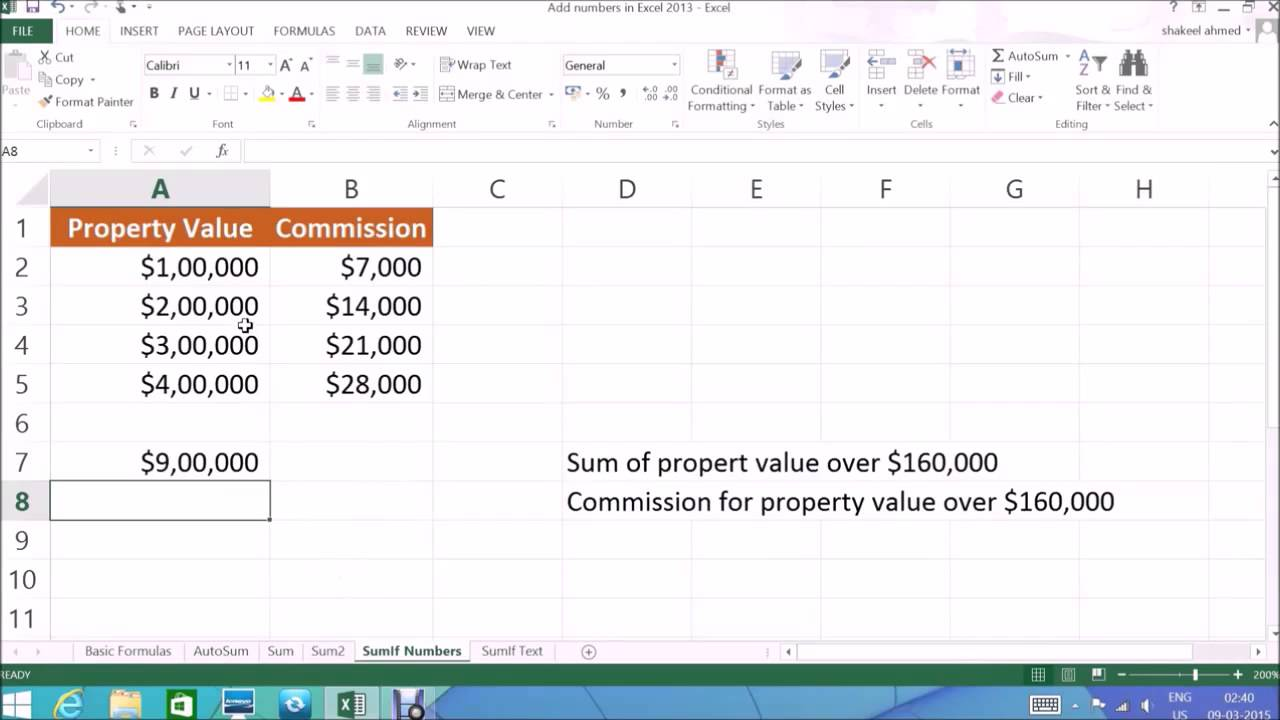## Excel Tips : SUM SUMIF & SUMIF using Text

Ever wondered how to calculate the Sum of a particular data values from your Total Sample or how to easily calculate the sum of all the adjacent Rows or columns. The Sum & Sum if functions are the ones best suitable for these kind of calculation and this video shows the easiest way to add using Sum & Sum if function.
This is part 2 of the video, below is the link of part one:

Terms & Definitions:
Function
A function is a predefined formula that helps us to save time
To create a formula with the SUM Function you start with =sign, SUM, select the range and press enter

Sumif Function
The Sumif function adds the values based on the criteria that we provide, for example if I want the total of property values which are over \$160,000 then we need to use Sumif.

Sumif Text
We can also evaluate text in Sumif function, we start with = sign, SUMif, open parenthesis, select the cell, put the criteria in Quotes, in this example fruits, select the sum range and then press enter and we see the total for fruit sales is \$4000.

To add the sales for food ending in es =sign, SUMIF, open parenthesis, select the range, comma, put the criteria in Quotes in this case *es, select the sum range and the enter